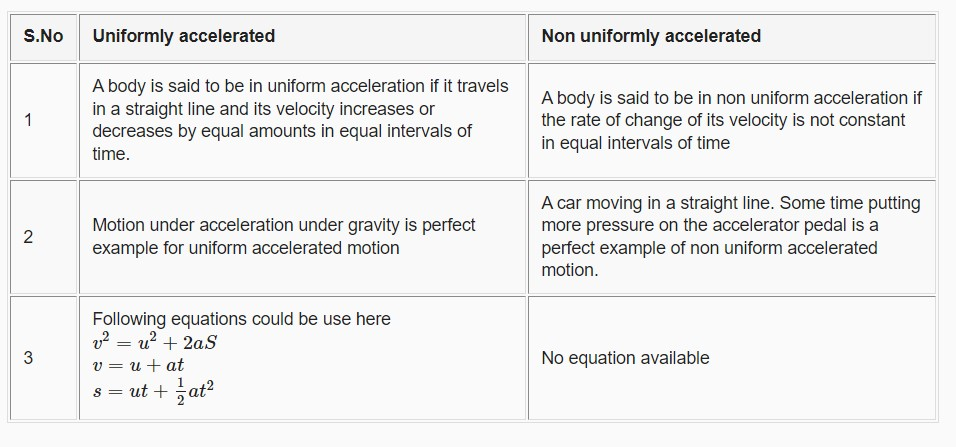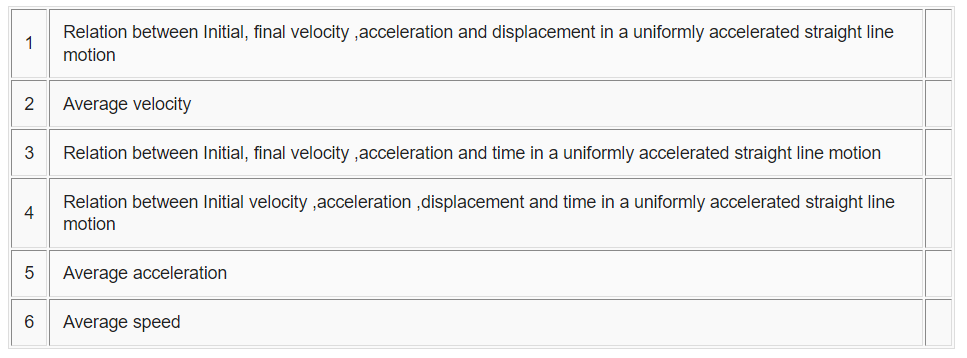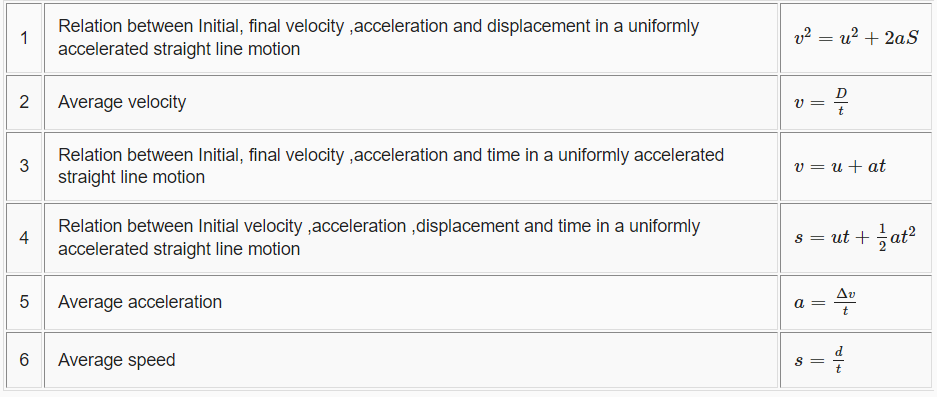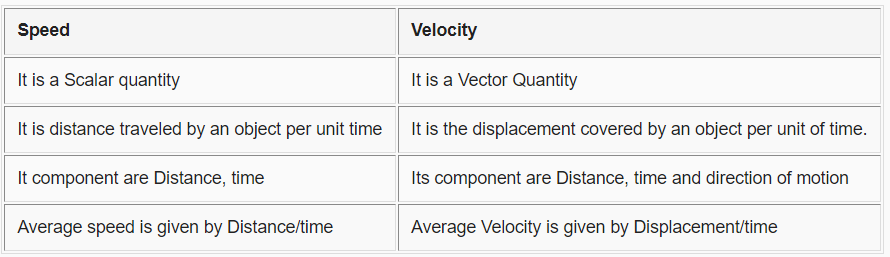# Conceptual Motion Problems and Solution

Given below are the CBSEConceptual Questions and answers on Motion for Class 9 physics
(a) Very Short questions
(b) True and false (c) Short questions
Hope you like them and do not forget to like , social share and comment at the end of the page.

## Very Short Answer Based questions for Motion

Question 1.
1. What is unit of acceleration?
2. What is zero uniform velocity motion?
3. A body moving in a circle with constant speed. Is it having acceleration?
4. A body goes from point A to B and then return then from B to A. what is the total displacement?
5. Acceleration is a vector quantity True or false?
6. What is the path of a velocity –time graph of a body moving with uniform acceleration
7. What all ways the velocity of the body can be changed?
8. What is average speed?

1. m/s2
2. It is the object at rest
3. Yes as the direction of the velocity is changing
4. Zero
5. True
6. Straight line
7. We can change either in magnitude or direction or we can change both the magnitude and direction
8. Average speed is the ratio of total distance travelled to the total time taken

## True and False

Question 2.
True and False Statements
A. Displacement can be zero but distance never
B. Displacement magnitude can be greater than distance travelled by the object
C. Time is a vector quantity
D. If the velocity of the body decreases with time, the acceleration is negative (retardation), and the motion is called decelerated motion
E. The area of the velocity time graph gives displacement of the body
F. Acceleration is a scalar quantity
G. Motion and rest are relative terms
I. an object can be moving with Uniform speed but variable acceleration

A. True
B. True
C. False
D. True
E. True
F. False
G. True
I. True

Question 3.
A object goes from point X to Y and then come back from Y to X. What is the displacement and average velocity?

Displacement is zero as it come back to it initial point.
Now Average velocity is given Displacement/time. Since displacement is zero, Average velocity is also zero

Question 4.
Write down the difference between Scalars and Vectors?

S.No Scalars Vectors
1 It has magnitude only It has magnitude and direction both
2 Example are speed,time,mass,charge Example are velocity,displacement,acceleration,force

Question 5.
Four Cyclist A,B,C ,D starts at the same point and at the same time and move in a straight line to reach destination .They all move with uniform velocities. They reach the destination in the following order
$C \to A \to B \to D$
a. If the displacement-time graph is plotted for each cyclist, which will be having highest slope
b. Arrange the cyclist in decreasing order of velocity

Since C is fastest cyclist, it velocity is highest and Slope of displacement time graph will be highest for him Velocity of cyclist in decreasing order
$C > A > B > D$

Question 6.
What do you infer if ?
 Distance –time graph is straight line 2 Velocity –time graph is curved 3 Displacement –time is zig zag

 Distance – time graph is straight line Speed is constant 2 Velocity – time graph is curved Acceleration is not uniform 3 Displacement – time is zigzag Non uniform velocity

Question 7.
What is the difference between Uniform Accelerated and Non Uniform accelerated motion?Question 8.
What happens to speed, velocity acceleration when an object moves in a circle with uniform speed?

Speed remains constant
Velocity magnitude remains constant, but direction changes continuously
Acceleration magnitude remains constant but direction changes continuously

Question 9.
When an object is thrown upwards, what is true of velocity and acceleration at the highest point of motion of the object?

Velocity becomes zero
Acceleration remains same as g

Question 10.
Gave the formula for eachQuestion 11.
Difference between Speed and Velocity?Question 12.
Explain why is difficult for a fireman to hold a house, which ejects large amounts of water at a high velocity?

Question 13.
Differentiate between uniform acceleration and non uniform acceleration? Define one Newton.

Question 14.
What happens when we accidentally step on a peel of banana? Explain with reference to Newton’s law of motion.

## Summary

This conceptual questions of physics class 9 chapter 8 is prepared keeping in mind the latest syllabus of CBSE . This has been designed in a way to improve the academic performance of the students. If you find mistakes , please do provide the feedback on the mail. You can also find the pdf for download